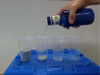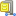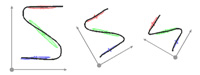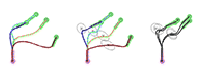### Abstract

Robot learning from demonstration faces new challenges when applied to tasks in which forces play a key role. Pouring liquid from a bottle into a glass is one such task, where not just a motion with a certain force profile needs to be learned, but the motion is subtly conditioned by the amount of liquid in the bottle. In this paper, the pouring skill is taught to a robot as follows. In a training phase, the human teleoperates the robot using a haptic device, and data from the demonstrations are statistically encoded by a parametric hidden Markov model, which compactly encapsulates the relation between the task parameter (dependent on the bottle weight) and the force-torque traces. Gaussian mixture regression is then used at the reproduction stage for retrieving the suitable robot actions based on the force perceptions. Computational and experimental results show that the robot is able to learn to pour drinks using the proposed framework, outperforming other approaches such as the classical hidden Markov models in that it requires less training, yields more compact encodings and shows better generalization capabilities.

### Bibtex reference

@inproceedings{Rozo13ROMOCO,
author="Rozo, L. and Jimenez, P. and Torras, C.",
title="Force-based Robot Learning of Pouring Skills using Parametric Hidden Markov Models",
booktitle="{IEEE-RAS} Intl Workshop on Robot Motion and Control",
year="2013",
pages="227--232"
}

### Video

Demonstration phase: The human provides examples of the pouring task by teleoperating the robotic arm using a haptic device. The force perceptions sensed over the course of the task, along with their initial value (i.e., the task parameter), are used to train a parametric hidden Markov model.

Robot execution stage: The robot carries out the pouring skill using Gaussian mixture regression to retrieve joint-level commands given the force-torque inputs at each time step.

NOTE: The execution carried out by the robot is slow and sometime also shows "jerkiness", this occurs because two aspects, namely, (i) the provided demonstrations showed low velocity profiles which do not vary smoothly, and (ii) the robot controller only provides a position controller, thus velocity control is not possible here in order to get smoother and more realistic reproductions.

### Source codes

Training of a task-parameterized Gaussian mixture model (GMM) based on candidate frames of reference. The proposed task-parameterized GMM approach relies on the linear transformation and product properties of Gaussian distributions to derive an expectation-maximization (EM) algorithm to train the model. The proposed approach is contrasted with an implementation of the approach proposed by Wilson and Bobick in 1999, with an implementation applied to GMM (that we will call PGMM) and following the model described in "Parametric Hidden Markov Models for Gesture Recognition", IEEE Trans. on Pattern Analysis and Machine Intelligence.
In contrast to the standard PGMM approach, the new approach that we propose allows the parameterization of both the centers and covariance matrices of the Gaussians. It has been designed for targeting problems in which the task parameters can be represented in the form of coordinate systems, which is for example the case in robot manipulation problems.Download task-parameterized GMM Matlab sourcecodeDownload task-parameterized GMM C++ (command line version) sourcecode

#### Usage

For the Matlab version, unzip the file and run 'demo1' or 'demo2' in Matlab.
For the C++ version, unzip the file and follow the instructions in the ReadMe.txt file.

#### Reference

• Calinon, S., Li, Z., Alizadeh, T., Tsagarakis, N.G. and Caldwell, D.G. (2012) Statistical dynamical systems for skills acquisition in humanoids. Proc. of the IEEE Intl Conf. on Humanoid Robots (Humanoids).

#### Demo 1 - Simple example of task-parameterized GMM learning and comparison with standard PGMMThis example uses 3 trajectories demonstrated in a frame of reference that varies from one demonstration to the other. A model of 3 Gaussian components is used to encode the data in the different frames, by providing the parameters of the coordinate systems as inputs (transformation matrix A and offset vector b).

#### Demo 2 - Example of task-parameterized movement learning with DS-GMR (statistical dynamical systems based on Gaussian mixture regression)This demo shows how the approach can be combined with the DS-GMR model to learn movements modulated with respect to different frames of reference. The DS-GMR model is a statistical dynamical system approach to learn and reproduce movements with a superposition of virtual spring-damper systems retrieved by Gaussian mixture regression (GMR). For more details, see the 'DMP-learned-by-GMR-v1.0' example code downloadable from the website below.